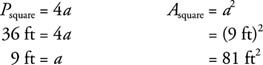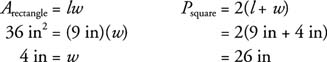## Squares and Rectangles

Perimeter refers to the entire length of a figure or the distance around it. If the figure is a circle, the length is referred to as the circumference. Such lengths are always measured in linear units such as inches, feet, and centimeters. Area refers to the size of the interior of a planar (flat) figure. Area is always measured in square units such as square inches (in2), square feet (ft2), and square centimeters (cm2), or in special units such as acres or hectares.

For all polygons, you find perimeter by adding together the lengths of all the sides. In this article, P is used to stand for perimeter, and A is used to stand for area.

## Finding the perimeter

Figures 5.1(a) and 5.1(b) show perimeter formulas for squares and rectangles.Figure 1 Perimeter of a square and perimeter of a rectangle.

Area formulas for squares and rectangles are formed by simply multiplying any pair of consecutive sides together. Refer to Figures 5.1(a) and 5.1(b).Example 1: Find the perimeter and area of Figure 2.Figure 2 Finding the perimeter and area of a square.

This is a square.Example 2: Find the perimeter and area of Figure 3.Figure 3 Finding the perimeter and area of a rectangle.

This is a rectangle.Example 3: If the perimeter of a square is 36 ft, find its area.The area of the square would be 81 square feet.

Example 4: If a rectangle with length 9 in has an area of 36 in 2, find its perimeter.The perimeter of the rectangle would be 26 inches.

Back to Top
A18ACD436D5A3997E3DA2573E3FD792A